## Symmetrical pi Network in Network Analysis:

The Symmetrical pi Network in Network Analysis is another important network in line transmission fulfilling the conditions of total series and shunt arm impedances as Z1 and Z2 respectively. Thus the series arm impedance of a it network is selected as Z1 and to have a total shunt arm impedance of Z2, each shunt arm impedance is selected as 2Z2 as shown in the Fig. 8.15.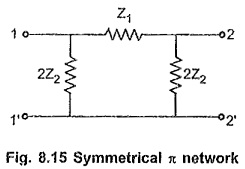Similar to the symmetrical T network, let us derive the expressions for the characteristic impedance (Z0) and propagation constant (γ) of the Symmetrical pi Network in Network Analysis.

### Characteristic Impedance (Z0):

(A) In terms of series and shunt arm impedances

Consider a symmetrical π network terminated at its output terminals with its characteristic impedance Z0 as shown in the Fig. 8.16.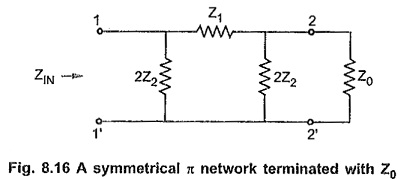By the property of the symmetrical network, the input impedance of such network terminated with Z0 at other port is equal to Z0.

The input impedance of a symmetrical π network is given by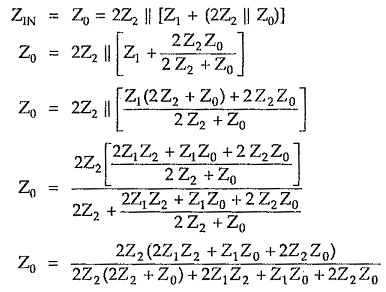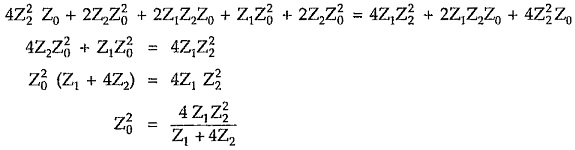Multiplying numerator and denominator by the factor Z1/4,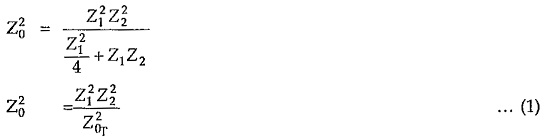Here the characteristic impedance of it section is indicated by Z and that of T section by Z0T.

Taking square root on both the sides,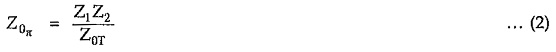(B) In terms of open and short circuit impedances

Consider Symmetrical pi Network in Network Analysis shown in the Fig. 8.17 (a) and Fig. 8.17 (b).

Consider Fig. 8.17 (a),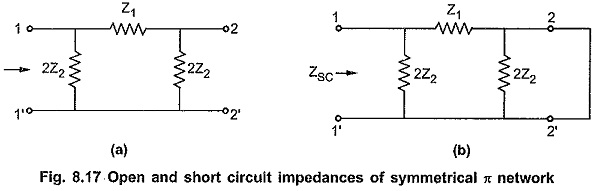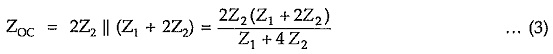Consider Fig. 8.17 (b),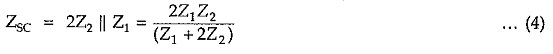Multiplying equations (3) and (4), we can write,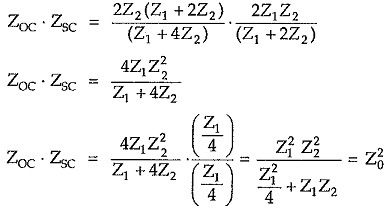Thus the characteristic impedance of symmetrical π network is given by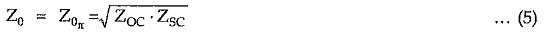### Propagation Constant (γ):

Consider correctly terminated symmetrical π network as shown in the Fig. 8.19.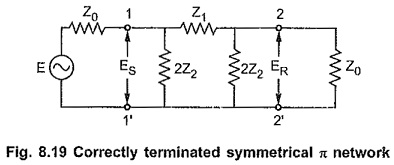As the network is symmetrical, by definition,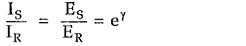By potential divider rule,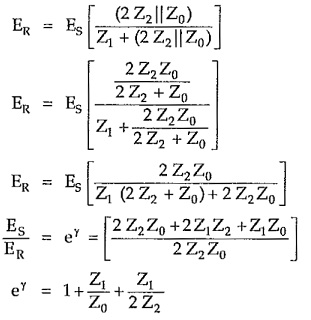Rearranging the terms,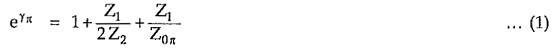where γπ is the propagation constant of symmetrical π network. Putting value of Z interms of Z0T,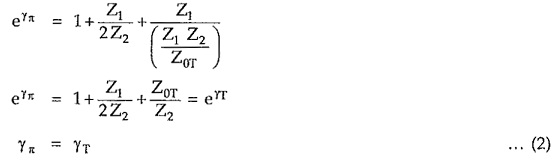Series and shunt arm impedances of symmetrical π network in terms of Z and γπ:

For symmetrical T network, each series arm is given by,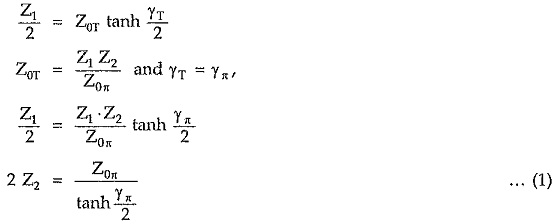Similarly shunt arm of symmetrical T network is given by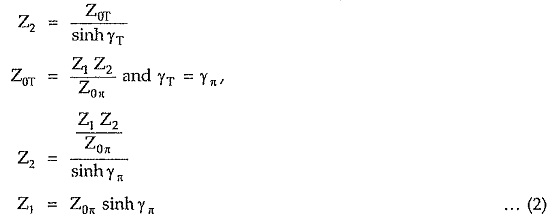Hence π network with series and shunt arms expressed in terms of Z and γπ, is as shown in the Fig. 8.20.

Scroll to Top